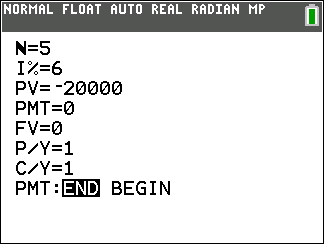### Mathematics lessons for IB® Diploma Programme

Applications and Interpretation | Numbers and Algebra# Compound Interest

### Activity Overview

This activity involves exploring the formula for compound interest as a function of the initial deposit, interest rate, and the number of pay periods per year.

### Objectives

• Students will interpret the variables in the formula for compound interest.
• Students will use the formula for compound interest and understand the effects of changes in the interest rate and the number of compounding periods.
• Students will understand the relationship between compound interest and continuous compounding

### Vocabulary

 compound interest interest rate pay periods initial deposit continuous compounding